Here you’ll learn about how to add fractions with like denominators and with unlike denominators. You will also learn how to add mixed numbers.

Students will first learn about adding fractions as part of number and operations in fractions in elementary school.

Adding fractions is when you combine two or more fractions together to find the total.

To do this, fractions need a common denominator (bottom number). Then you can add the fractions by adding the numerators (top numbers).

For example,

Since the denominators are the same, you need to add the numerators 3+7=10.

There are 10 parts. But what size are the parts? They are still eighths, so the denominator stays the same and the answer is 10 eighths, or 1 and 2 eighths

You can also write this answer as the equivalent fraction 1\cfrac{1}{4}.

If the denominators are not the same, you create equivalent fractions before adding.

For example,

Since the denominators are NOT the same, the parts are NOT the same size. Use equivalent fractions to create a common denominator of 9. Multiply the numerator and denominator of \cfrac{2}{3} by 3.

\cfrac{2\times3}{3\times3}=\cfrac{6}{9}

You add to see how many parts there are in total: 6+5=11.

There are 11 parts. But what size are the parts? They are still ninths, so the denominator stays the same and the answer is 11 ninths, or 1 and 2 ninths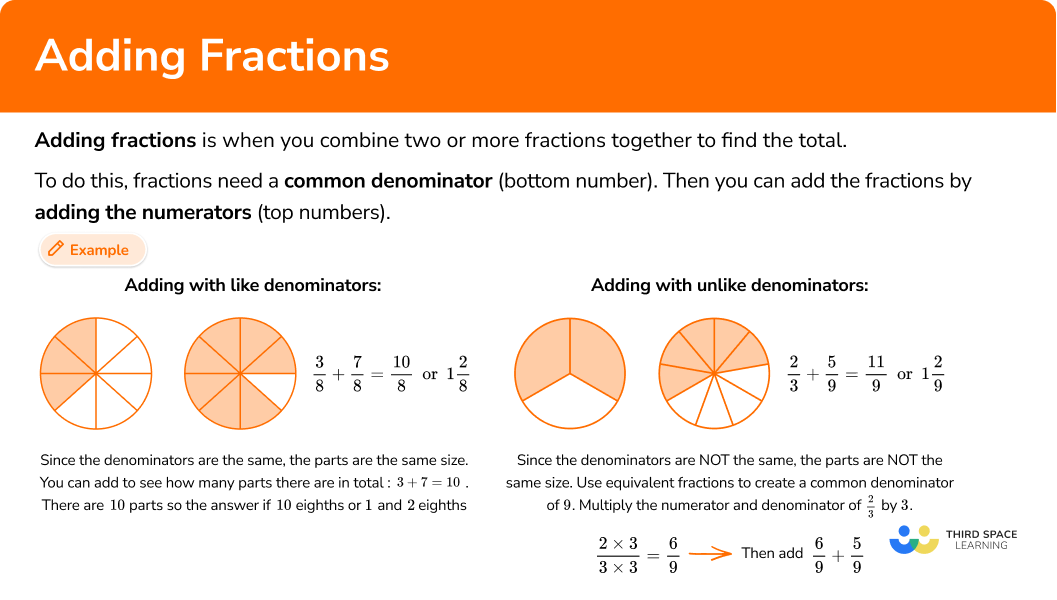## Common Core State Standards

How does this relate to 4th grade math and 5th grade math?

• Grade 4 – Number and Operations – Fractions (4.NF.B.3c)
Add and subtract mixed numbers with like denominators, e.g., by replacing each mixed number with an equivalent fraction, and/or by using properties of operations and the relationship between addition and subtraction.
• Grade 5 – Number and Operations – Fractions (5.NF.A.1)
Add and subtract fractions with unlike denominators (including mixed numbers) by replacing given fractions with equivalent fractions in such a way as to produce an equivalent sum or difference of fractions with like denominators.
For example, \cfrac{2}{3}+\cfrac{5}{4}=\cfrac{8}{12}+\cfrac{15}{12}=\cfrac{23}{12}. (In general, \cfrac{a}{b}+\cfrac{c}{d}=\cfrac{(ad+bc)}{bd}. )

In order to add fractions with like denominators:

1. Add the numerators (top numbers).

In order to add mixed numbers with like denominators:

In order to add fractions with unlike denominators:

1. Create common denominators (bottom numbers).
2. Add the numerators (top numbers).

In order to add mixed numbers with unlike denominators:

2. Create common denominators (bottom numbers).

### Example 1: adding fractions with like denominators

Solve \cfrac{3}{5}+\cfrac{4}{5}.

1. Add the numerators (top numbers).

Since the denominators are the same, the parts are the same size. You add to see how many parts there are in total: 3+4=7.

There are 7 parts. But what size are the parts? They are still fifths, so the denominator stays the same.

\cfrac{3}{5}+\cfrac{4}{5}=\cfrac{7}{5} \ or \ 1 \cfrac{2}{5}

### Example 2: adding mixed numbers with like denominators

Solve 4\cfrac{5}{6}+1\cfrac{2}{6}.

Since the denominators are the same, the parts are the same size. You add to see how many parts there are in total: 5+2=7.

There are 7 parts. But what size are the parts? They are still sixths, so the denominator stays the same.

\cfrac{5}{6}+\cfrac{2}{6}=\cfrac{7}{6} \ or \ 1 \cfrac{1}{6}

Add the whole numbers and fraction together.

### Example 3: adding mixed numbers with like denominators

Solve 3 \cfrac{7}{10}+3 \cfrac{5}{10}.

Since the denominators are the same, the parts are the same size. You add to see how many parts there are in total: 5+7=12.

There are 12 parts. But what size are the parts? They are still tenths, so the denominator stays the same.

\cfrac{7}{10}+\cfrac{5}{10}=\cfrac{12}{10} \ or \ 1 \cfrac{2}{10}

Add the whole numbers and fraction together.

You can also write this answer as the equivalent fraction 7 \cfrac{1}{5}.

### Example 4: adding fractions with unlike denominators

Solve \cfrac{4}{10}+\cfrac{1}{3}.

Since \cfrac{4}{10} and \cfrac{1}{3} do not have like denominators, the parts are NOT the same size. Use equivalent fractions to create a common denominator. Multiply each fraction by the opposite denominator.

Since the denominators are the same, the parts are the same size. You add to see how many parts there are in total: 12+10=22.

There are 22 parts. But what size are the parts? They are still thirtieths, so the denominator stays the same.

You can also write this answer as the equivalent fraction \cfrac{11}{15}.

### Example 5: adding mixed numbers with unlike denominators

Solve 2 \cfrac{3}{8}+1 \cfrac{7}{12}.

Since \cfrac{3}{8} and \cfrac{7}{12} do not have like denominators, the parts are NOT the same size. Use equivalent fractions to create a common denominator.

A common denominator of 24 can be used. Multiply the numerator and denominator of each fraction by the factor that will create a denominator of 24.

\cfrac{3}{8}=\cfrac{3 \times 3}{8 \times 3}=\cfrac{9}{24} \ and \ \cfrac{7}{12}=\cfrac{7 \times 2}{12 \times 2}=\cfrac{14}{24}

Since the denominators are the same, the parts are the same size. You add to see how many parts there are in total: 9+14=23.

There are 23 parts. But what size are the parts? They are still twenty-fourths, so the denominator stays the same.

\cfrac{9}{24}+\cfrac{14}{24}=\cfrac{23}{24}

Add the whole number and fraction together.

### Example 6: adding mixed numbers with unlike denominators

Solve 4 \cfrac{2}{3}+5 \cfrac{5}{6}.

Since \cfrac{2}{3} and \cfrac{5}{6} do not have like denominators, the parts are NOT the same size. Use equivalent fractions to create a common denominator.

A common denominator of 6 can be used. Multiply the numerator and denominator of \cfrac{2}{3} by the factor that will create a denominator of 6.

\cfrac{2}{3}=\cfrac{2 \times 2}{3 \times 2}=\cfrac{4}{6} \ and \ \cfrac{5}{6}

Since the denominators are the same, the parts are the same size. You add to see how many parts there are in total: 4+5=9.

There are 9 parts. But what size are the parts? They are still sixths, so the denominator stays the same.

\cfrac{4}{6}+\cfrac{5}{6}=\cfrac{9}{6} or 1 \cfrac{3}{6}

Add the whole numbers and fraction together.

You can also write this answer as the equivalent mixed number 10 \cfrac{1}{2}.

### Teaching tips for adding fractions

• Fraction work in 3rd grade centers around understanding through models, particularly area models and number lines. To build on this in 4th grade and 5th grade, always have physical or digital models available for students to use when necessary.

• To introduce this topic, give students a fraction addition problem and give them time to solve in a way that makes sense to them. Then go over the different solving strategies as a whole group.

• In the beginning, you may want to stick to smaller fractions or problems that do not involve regrouping. However, at some point it is important to transition to showing students all kinds of fraction addition problems mixed together. This gives them an opportunity to identify which solving strategy to use.

• Fraction worksheets may have their place when students are still developing understanding around addition, but once students have a successful strategy and can flexibly operate, incorporate math games or real world projects that involve addition of fractions.

### Our favorite mistakes

When fractions are added together, they must have like denominators. Before adding fractions with unlike denominators, use equivalent fractions to create a common denominator.

The denominator tells us how big the parts are. You add the number of parts (numerators) and keep the denominator (the size of the parts) the same.

1. Solve \cfrac{8}{12}+\cfrac{9}{12}.

\cfrac{17}{24}1 \cfrac{5}{12}1 \cfrac{5}{24}\cfrac{1}{12}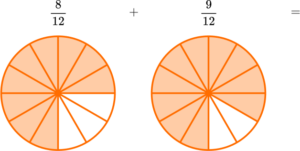Since the denominators are the same, the parts are the same size. You add to see how many parts there are in total: 8+9=17.

There are 17 parts. But what size are the parts? They are still twelfths, so the denominator stays the same.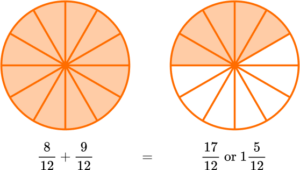2. Solve 2 \cfrac{4}{8}+2 \cfrac{7}{8}.

4 \cfrac{11}{16}5 \cfrac{3}{16}4 \cfrac{1}{8}5 \cfrac{3}{8}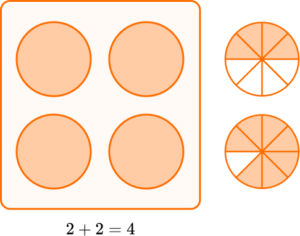Since the denominators are the same, the parts are the same size. You add to see how many parts there are in total: 4+7=11.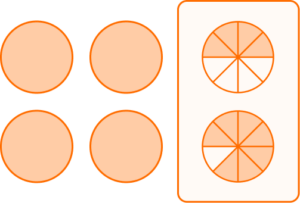There are 11 parts. But what size are the parts? They are still eighths, so the denominator stays the same.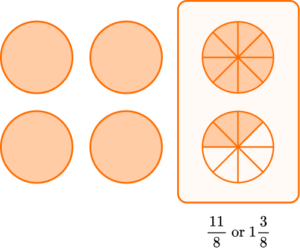\cfrac{4}{8}+\cfrac{7}{8}=\cfrac{11}{8} \ or \ 1 \cfrac{3}{8}

Add the whole numbers and fraction together.3. Solve 1 \cfrac{2}{5}+7 \cfrac{4}{5}.

9 \cfrac{1}{5}8 \cfrac{6}{10}8 \cfrac{1}{5}9 \cfrac{1}{10}Since the denominators are the same, the parts are the same size. You add to see how many parts there are in total: 2+4=6.There are 6 parts. But what size are the parts? They are still fifths, so the denominator stays the same.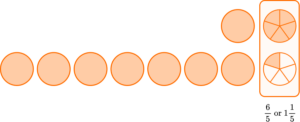\cfrac{2}{5}+\cfrac{4}{5}=\cfrac{6}{5} \ or \ 1 \cfrac{1}{5}

Add the whole numbers and fraction together.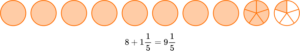4. Solve \cfrac{3}{4}+\cfrac{10}{12}.

\cfrac{13}{16}1 \cfrac{7}{16}1 \cfrac{7}{12}\cfrac{13}{12}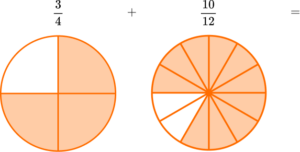Since \cfrac{3}{4} and \cfrac{10}{12} do not have like denominators, the parts are NOT the same size. Use equivalent fractions to create a common denominator.

A common denominator of 12 can be used. Multiply the numerator and denominator of \cfrac{3}{4} by the factor that will create a denominator of 12.

\cfrac{3}{4}=\cfrac{3 \times 3}{4 \times 3}=\cfrac{9}{12} \ and \ \cfrac{10}{12}Since the denominators are the same, the parts are the same size. You add to see how many parts there are in total: 9+10=19.

There are 19 parts. But what size are the parts? They are still twelfths, so the denominator stays the same.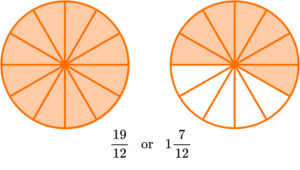\cfrac{9}{12}+\cfrac{10}{12}=\cfrac{19}{12} \ or \ 1 \cfrac{7}{12}

5. Solve 6 \cfrac{1}{2}+1 \cfrac{3}{10}.

7 \cfrac{8}{10}7 \cfrac{4}{12}8 \cfrac{8}{12}7 \cfrac{4}{20}Since \cfrac{1}{2} and \cfrac{3}{10} do not have like denominators, the parts are NOT the same size. Use equivalent fractions to create a common denominator.

A common denominator of 10 can be used. Multiply the numerator and denominator of \cfrac{1}{2} by the factor that will create a denominator of 10.

\cfrac{1}{2}=\cfrac{1 \times 5}{2 \times 5}=\cfrac{5}{10} \ and \ \cfrac{3}{10}Since the denominators are the same, the parts are the same size. You add to see how many parts there are in total: 5+3=8.

There are 8 parts. But what size are the parts? They are still tenths, so the denominator stays the same.Add the whole number and fraction together.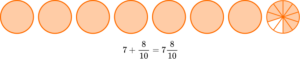6. Solve 3 \cfrac{5}{6}+4 \cfrac{3}{5}.

7 \cfrac{8}{11}8 \cfrac{13}{60}7 \cfrac{8}{30}8 \cfrac{13}{30}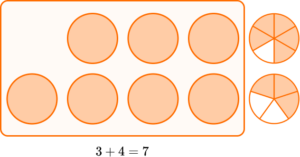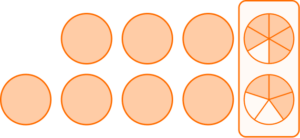Since \cfrac{5}{6} and \cfrac{3}{5} do not have like denominators, the parts are NOT the same size. Use equivalent fractions to create a common denominator.

Multiplying the numerator and denominator by the opposite denominator will create common denominators.

\cfrac{5}{6}=\cfrac{5 \times 5}{6 \times 5}=\cfrac{25}{30} \ and \ \cfrac{3}{5}=\cfrac{3 \times 6}{5 \times 6}=\cfrac{18}{30}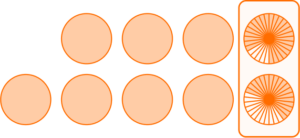Since the denominators are the same, the parts are the same size. You add to see how many parts there are in total: 25+18=43.

You have 43 parts. But what size are the parts? They are still thirtieths, so the denominator stays the same.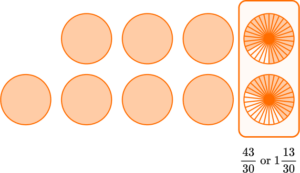\cfrac{25}{30}+\cfrac{18}{30}=\cfrac{43}{30} \ or \ 1 \cfrac{13}{30}

Add the whole numbers and fraction together.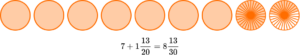Does multiplying by a common factor change the size of the fraction?

No, although a new numerator and new denominator are created the value of the fraction remains the same. Since the new fraction has a larger denominator, the parts will be smaller. The numerator also needs to be larger so that the total area of the parts is the same as the original fraction.

Do all fraction sums need to be in lowest terms?

No, students do not have to find the least common denominator in order to correctly answer a fraction addition question. However, as students progress in their understanding of fractions, it is a good habit to develop. It is also important to be mindful of standard expectations, as they may vary from state to state.

Is subtraction of fractions similar to addition of fractions?

Yes, you follow many of the same steps to subtract fractions, which also requires common denominators. The only difference is that you subtract the numerators instead of adding.

## Still stuck?

At Third Space Learning, we specialize in helping teachers and school leaders to provide personalized math support for more of their students through high-quality, online one-on-one math tutoring delivered by subject experts.

Each week, our tutors support thousands of students who are at risk of not meeting their grade-level expectations, and help accelerate their progress and boost their confidence.